# Discretization Invariant Learning on Neural Fields

Neural fields (NFs) like NeRF and SIREN are powerful representations of continuous data, but there’s a need for deep learning architectures that perform inference on such data without being sensitive to how the neural field is sampled, a property called discretization invariance.

We propose DI-Net, a general framework for discretization invariant learning on neural fields. DI-Net layers map NFs to NFs using the quasi-Monte Carlo method: they sample the input along a low discrepancy sequence and perform numerical integration with respect to some parametric map.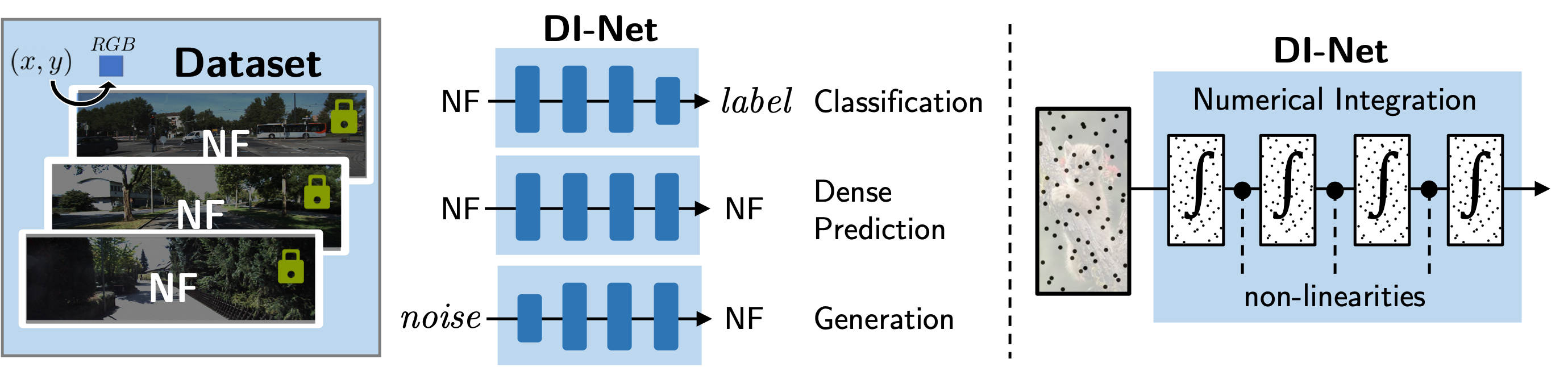Choosing a low discrepancy sequence minimizes the error between the discrete sample mean and the continuous integral. The parametric map can take on many familiar forms such as convolutions, attention and pooling.

DI-Nets generalize many existing network families as they bridge discrete and continuous network classes, such as convolutional neural networks (CNNs) and neural operators. They have desirable theoretical properties such as universal approximation of a large class of maps between $L^2$ functions of bounded variation, and gradients that are also discretization invariant.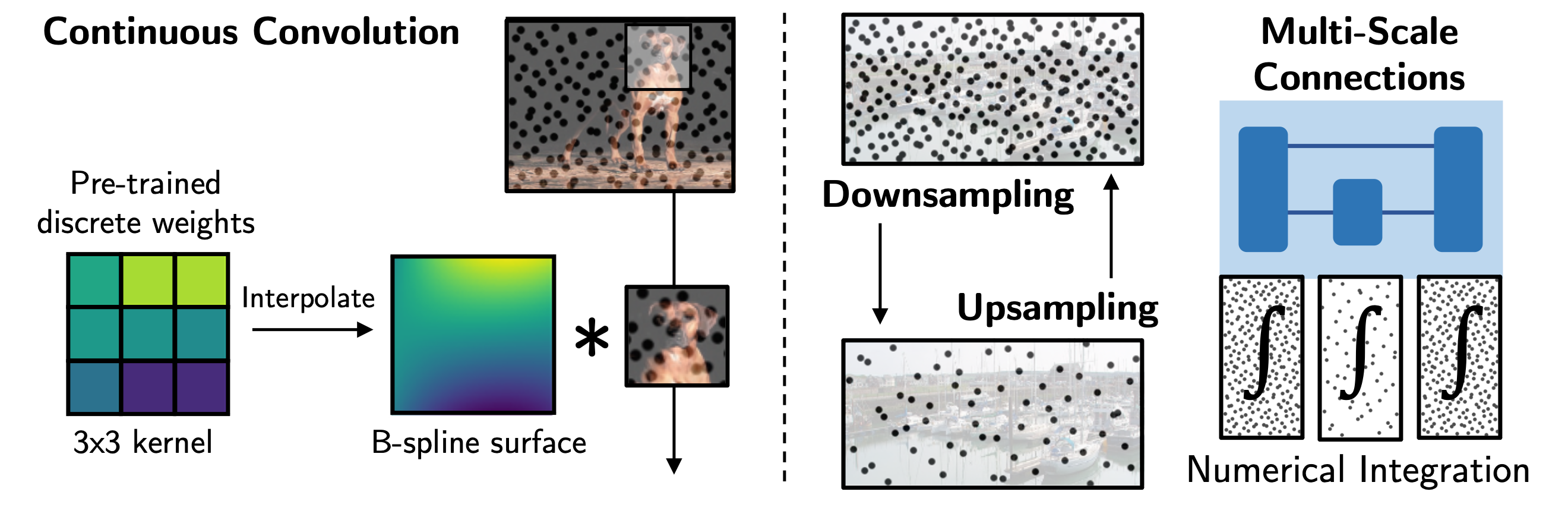DI-Nets derived from CNNs can learn tasks on neural fields under various discretizations, and often generalize to new types of discretizations at test time.

## Signed Distance Function Prediction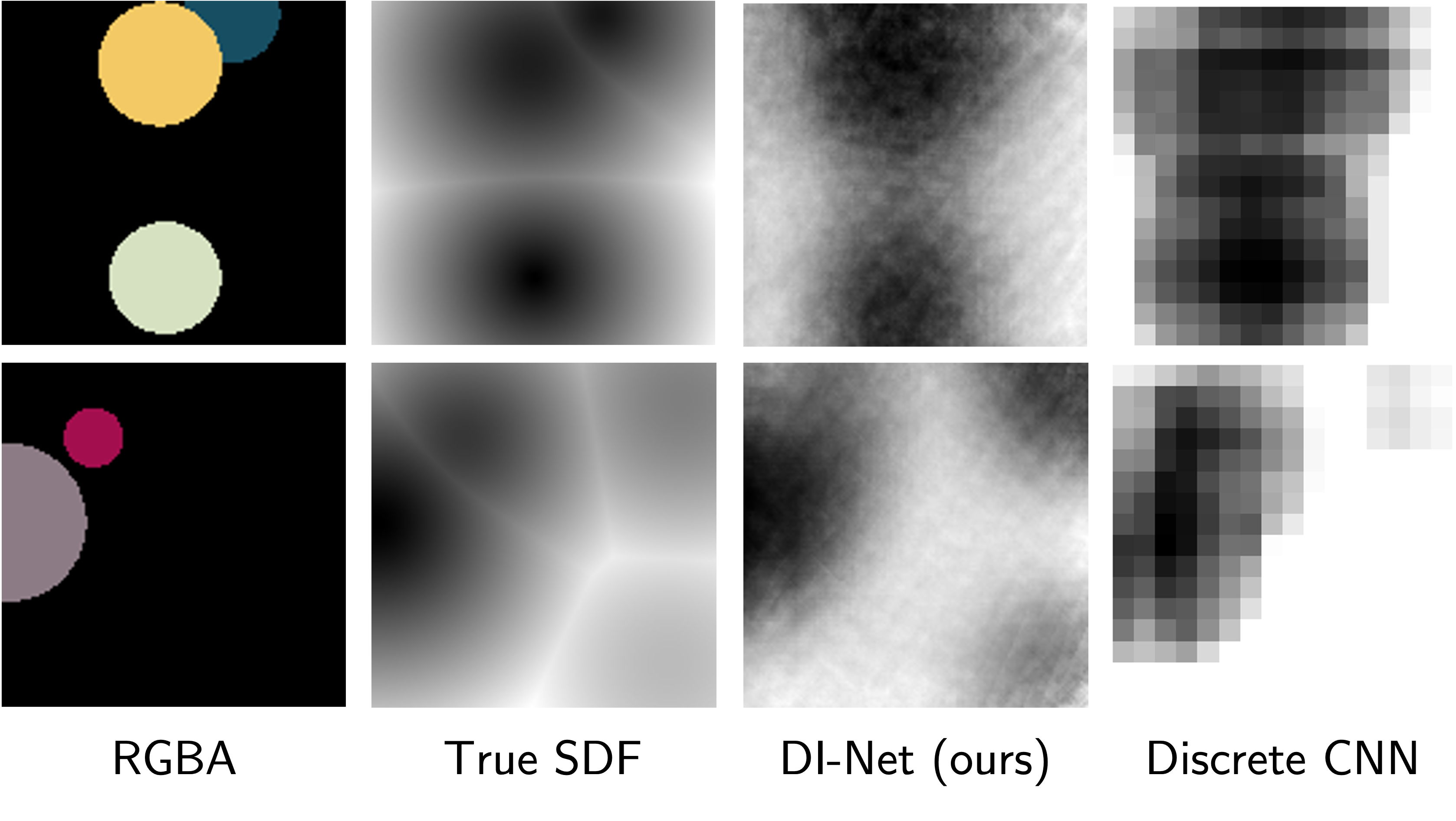## Segmentation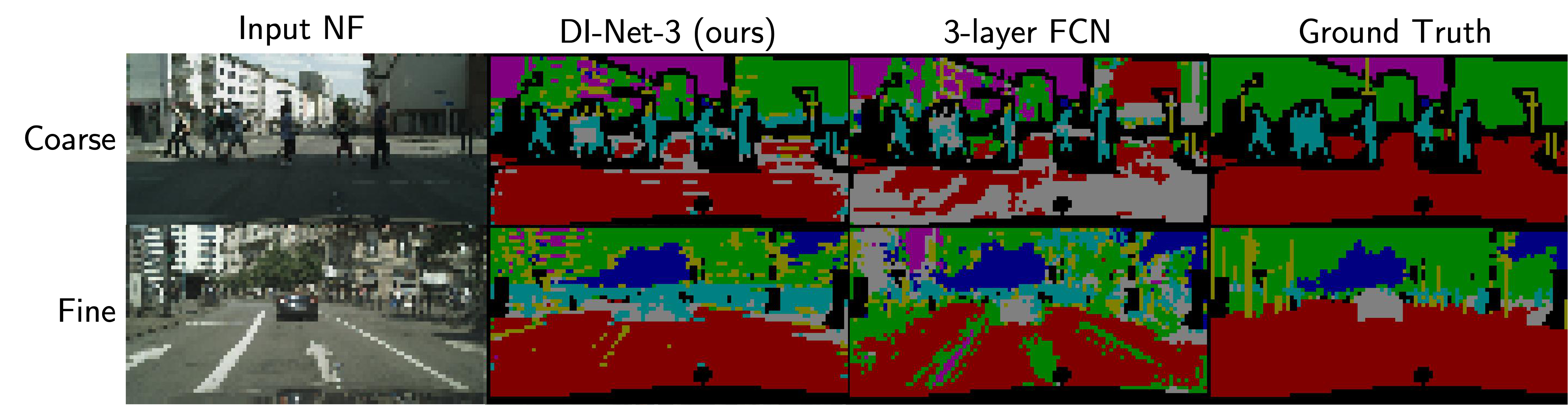## Classification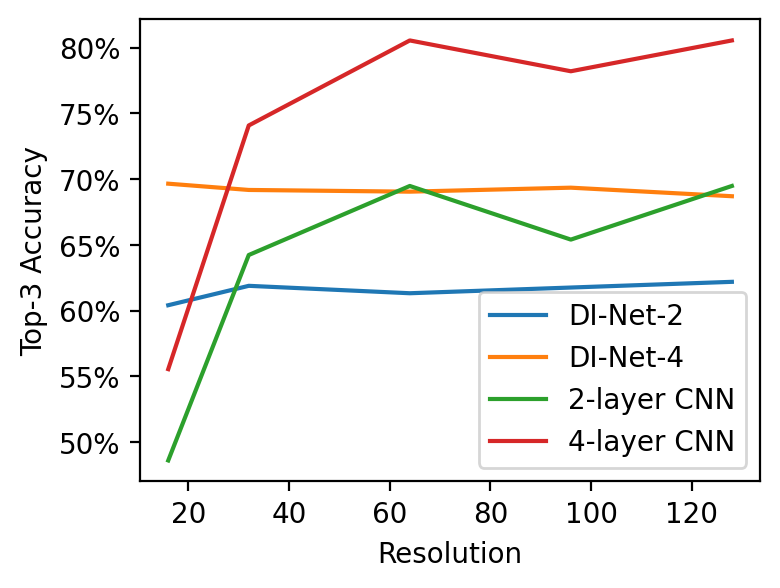Train $\to$ Test DiscretizationAccuracy
QMC $\to$ QMC32.9%
Grid $\to$ Grid30.5%
Shrunk $\to$ Shrunk30.3%
QMC $\to$ Grid27.1%
Grid $\to$ QMC27.8%
QMC $\to$ Shrunk25.4%
Shrunk $\to$ QMC13.4%

Read our paper for more details, or check out our code

### Bibtex

@misc{wang2022dinet,
title={Approximate Discretization Invariance for Deep Learning on Implicit Neural Datasets},
author={Clinton J. Wang and Polina Golland},
year={2022},
eprint={2206.01178},
archivePrefix={arXiv},
primaryClass={cs.LG}
}